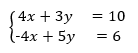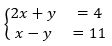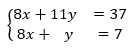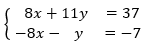# A.2.14.3 Adding and Subtracting Equations to Solve Systems

Topic:
Equations
Below are three systems of equations you saw earlier. For each system: 1. Use graphing technology to graph the original two equations in the system. Then, identify the coordinates of the solution. 2. Find the sum of the two original equations that would enable the system to be solved.   3. Graph the third equation on the same coordinate plane. Make an observation about the graph.

First system of equations:Second system of equations:Third system of equations:"Equivelant form" for this system: